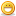1. ##100% in Life...

How does one achieve 100% in LIFE?
Begin by noting the following.

IF:
A = 1
B = 2
C = 3
D = 4
E = 5
F = 6
G = 7
H = 8
I = 9
J = 10
K = 11
L = 12
M = 13
N = 14
O = 15
P = 16
Q = 17
R = 18
S = 19
T = 20
U = 21
V = 22
W = 23
X = 24
Y = 25
Z = 26

Then:

H A R D W O R K = 8+1+18+4+23+15+18+11 = Only 98%

Similarly,

K N O W L E D G E = 11+14+15+23+12+5+4+7+5 = Only 96%

But interesting (and as you'd expect),
A T T I T U D E = 1+20+20+9+20+21+4+5 = 100% ...

This is how you achieve 100% in LIFE.

But even more interesting..

B U L L S H I T = 2+21+12+12+19+8+9+20 = 103%

So now you know what all those high-priced consultants, upper management, and motivational speakers really mean when they want to exceed 100%!2.3. ##good one..

hey good post man.....great keep it up...

intruder....4. LOL5. Now that´s logic!
Where I work the consultants cash in a lot and for doing one thing and that´s bullshitting.6. hehe... Thats pretty good####Posting Permissions

• You may not post new threads
• You may not post replies
• You may not post attachments
• You may not edit your posts
•